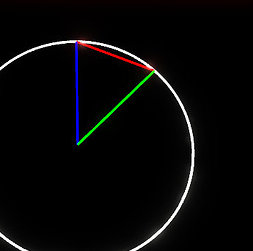# Whats the formula to this "function"?

This question is mostly math related…

How do I create this function WITHOUT using `Instance.new()` or anything outside of a script.

Here the red line is glitched into the green one, its the diameter of the circle.

As you can see it returns the length of the `red line` with the use of `θ` where the circle’s radius is `1`

if anyone knows whats the formula to the function please lmk :)))

2 Likes

you probably cant if it not parts or whatever is this

if its parts or Ui element then you can use position and size to determen the position and then CFrame (if parts) to look at eachother and then apply size

and thats just for only red line.
for green and blue. u set blue as it stays for center aka 0
and rotate green at the center first and then adding position that will make green line not in center but at its position like the exampleI mean i want to create a function just inside a script to achieve it.
like

``````function f(x)
🤔
end

print(f(90)) --> sqrt(2) (lazy to write it out)
print(f(180)) --> 2
print(f(45)) --> sqrt(1/2) (lazyyyy)
``````

Maybe you can take a look at this post:

i told you how but we cant write code for people thats againts the rules…

but for the rotation use math.rad

Ik but i need it to not use instances

im not sure if you can even make something without instances because its like everywhere

even the script is an instance :Ti meant the script shouldnt use instances.

like sin, cos, tan, cot, sec, csc is possible, why isnt this?

If you don’t want to use Instance.new() you have 2 choices:

1. take an existing instance and base your calculs over it
2. take defined numbers and base your calcul over it
1 Like

But like… what numbers do i use…?

What numbers should be used for this function?

like to get a circumference u do `f(x)=x*π`

so u use pi there

what do i use here…

You can do something like:

``````local function Calculation(x)
f(x)=x*π
end

print(Calculation(15))
``````

that doesnt make any sense…

f(x)=x*π ← that is a mathematical function, not a lua function

It was just an example to see how to do a function without using any instance. After that, you will have to create yourself the calcul inside

``````local function Calculation(x)
local pi = 3.14
x = x ^ 2
x = x * pi
return x

end

print(Calculation(15))
``````

first, this code is straight up bull___t.

...

pi already has a built in version, `math.pi`

x^2 is an existing function and you cant set what it results as.

this would print ` ` everytime because you didnt return anything.

I dont really think you understood my question…

I gave an example and yeah, i added a return now (just forgot it). have a good day